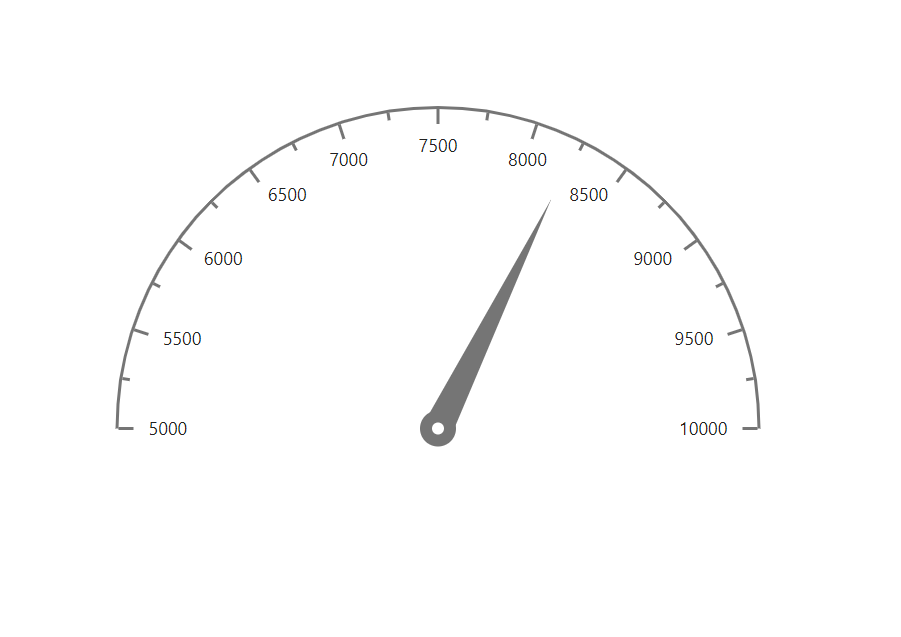Search results

# How To

21 Sep 2021 / 2 minutes to read

## Get data from database for circular gauge and render gauge from code behind

Circular gauge can be rendered from the code behind by initializing the required properties in controller and passing them through ViewData to client-side. You can get the data from the database by creating a data table and accessing the data table values to gauge pointers value.

To get the data from data base and render the gauge from code behind, follow the given steps:

Step 1:

Initialize the required properties in controller and get data from database, create a new data collection that contains minimum, maximum, and value attributes from the data base data, and then assign the data to the minimum, maximum, and pointer values of gauge.

`````` public ActionResult Index()
{
//Initialize the chart model
CircularGauge gaugeModel = new CircularGauge();
// Add the required properties for chart using the following method
InitializeGauge(gaugeModel);
// Store the chart model
ViewData["GaugeModel"] = gaugeModel;
return View();
}
private void InitializeGauge(CircularGauge gaugeModel)
{
// Create a data base data table
DataTable dt = new DataTable();
dt.Rows.Add(new Object[] { "T13", 10000, 5000, 8230 });

// Create  a new data collection from data base table
GaugeData gauges = new GaugeData();
foreach (DataRow row in dt.Rows)
{
gauges.Minimum = Convert.ToDouble(row["FreeStock"]);
gauges.Maximum = Convert.ToDouble(row["MaxStock"]);
gauges.Value = Convert.ToDouble(row["AvailableStock"]);
}

CircularGaugePointer pointer = new CircularGaugePointer();
pointer.Value = gauges.Value;

CircularGaugeAxis axis = new CircularGaugeAxis();
axis.Pointers = new List<CircularGaugePointer>();
axis.Minimum = gauges.Minimum;
axis.Maximum = gauges.Maximum;

gaugeModel.Axes = new List<CircularGaugeAxis>();
``   @Html.EJS().CircularGauge("container", (Syncfusion.EJ2.CircularGauge.CircularGauge)ViewData["GaugeModel"]).Render();``